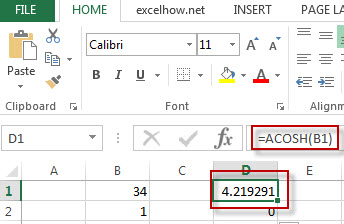# Excel ACOSH Function

This post will guide you how to use Excel ACOSH function with syntax and examples in Microsoft excel.

### Description

The Excel ACOSH function returns the inverse hyperbolic cosine of a number.

The ACOSH function is a build-in function in Microsoft Excel and it is categorized as a Math and Trigonometry Function.

The ACOSH function is available in Excel 2016, Excel 2013, Excel 2010, Excel 2007, Excel 2003, Excel XP, Excel 2000, Excel 2011 for Mac.

### Syntax

The syntax of the ACOSH function is as below:

= ACOSH (number)

Where the ACOSH function argument is:
Number – This is a required argument.  Any real number greater than or equal to 1.

### Example

The below example will show you how to use Excel ACOSH function to return the inverse hyperbolic cosine of the B1 cell.

#1 =ACOSH(B1)Note: the above formula will return the arccosine value of B1 cell.